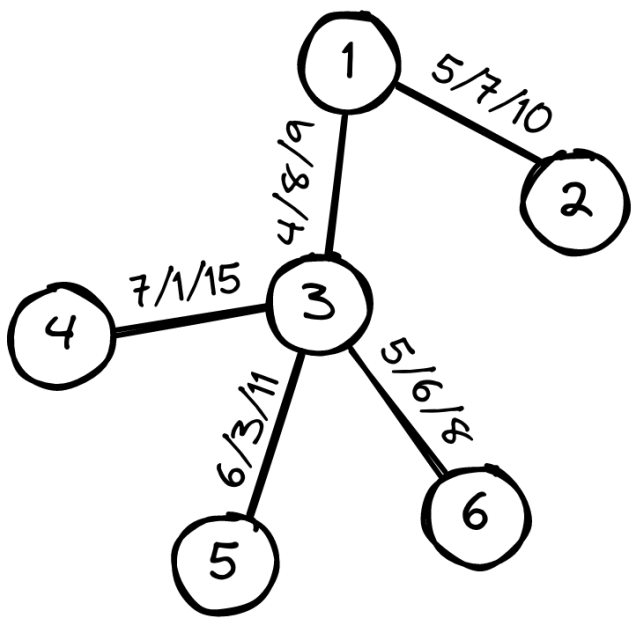시간 제한메모리 제한제출정답맞힌 사람정답 비율
5 초 1024 MB222100.000%

## 문제

The city mayor Mirko lives in a city with $n$ neighborhoods connected with $n - 1$ bidirectional roads such that from any neighborhood it is possible to reach every other neighborhood.

Mirko wants to upgrade some roads to reduce traffic. For every road, we know the current speed $v_i$ vehicles drive on it, the price of upgrading $c_i$ and the speed of driving after upgrading $s_i$.

There are $q$ unsatisfied citizens that come to visit Mirko. Each one has their suggestion for an upgrade. The suggestion of the $i$-th citizen is: “We should invest $e_i$ euros in upgrading roads between neighborhoods $a_i$ and $b_i$”

For each suggestion, Mirko is interested in what is the minimum driving speed between neighborhoods $a_i$ and $b_i$ if he spends at most $e_i$ euros on upgrading the roads, given thathis goal is to maximize the minimum driving speed between the neighborhoods $a_i$ and $b_i$.

Mirko soon realized that calculating this is not an easy task and hired you to help him!

## 입력

The first line contains the integer $n$ ($2 ≤ n ≤ 100\,000$), the number of neighborhoods.

In each of the next $n - 1$ lines there are five integers $x_i$, $y_i$, $v_i$, $c_i$, $s_i$ ($1 ≤ x_i , y_i ≤ n$, $1 ≤ v_i < s_i ≤ 10^9$, $1 ≤ c_i ≤ 10^9$), denoting that neighborhood $x_i$ and $y_i$ are connected, current driving speed is $v_i$, cost of upgrading the road is $c_i$, and the speed on the road would be $s_i$.

The next line contains the integer $q$ ($1 ≤ q ≤ 100\,000$), the number of unsatisfied citizens.

In each of the next $q$ lines there are three integers $a_i$, $b_i$, $e_i$ ($1 ≤ a_i , b_i ≤ n$, $a_i \ne b_i$, $1 ≤ e_i ≤ 10^{18}$), which describe the suggestion of the $i$-th citizen.

## 출력

In the $i$-th of the $q$ lines print the answer to the request of the $i$-th citizen.

## 서브태스크

번호배점제한
121

$n, q ≤ 1\,000$

229

Each of the neighborhoods will be connected with at most $2$ other neighborhoods.

360

## 예제 입력 1

6
1 2 5 7 10
1 3 4 8 9
3 4 7 1 15
3 5 6 3 11
3 6 5 6 8
3
2 4 15
6 4 5
3 5 10


## 예제 출력 1

7
5
11


## 예제 입력 2

4
1 2 5 5 8
2 3 4 6 9
3 4 6 10 7
4
1 4 16
2 4 16
1 4 10
3 4 10


## 예제 출력 2

6
7
5
7


## 힌트

Clarification of the first example:

The illustration represents the city and its neighborhoods. On the edges are written the current driving speed, the cost of upgrading, and the speed after upgrading, respectively.

If we upgrade the roads between $1$ and $2$, and between $1$ and $3$, the driving speeds from $2$ to $4$ will be $10$, $9$, and $7$ m/s. The minimum is $7$ m/s.

If we upgrade the roads between $4$ and $3$, the driving speeds from $6$ to $4$ will be $5$ an $15$ m/s. The minimum is $5$ m/s.

If we upgrade the road between $3$ and $5$, the driving speed from $5$ to $3$ will be $11$ m/s.## 채점 및 기타 정보

• 예제는 채점하지 않는다.